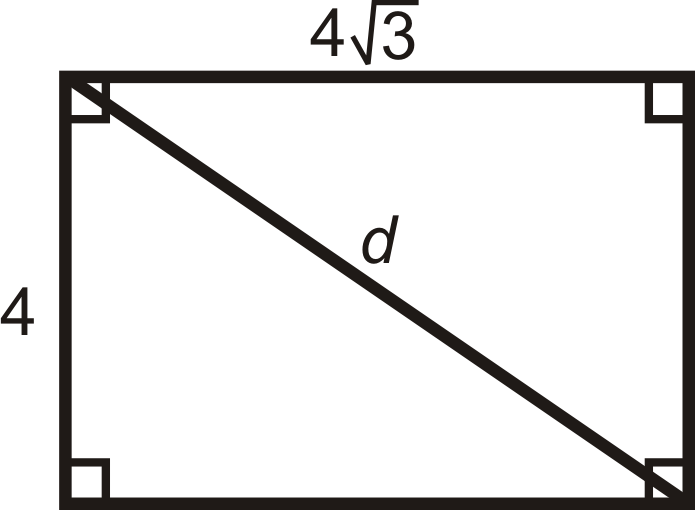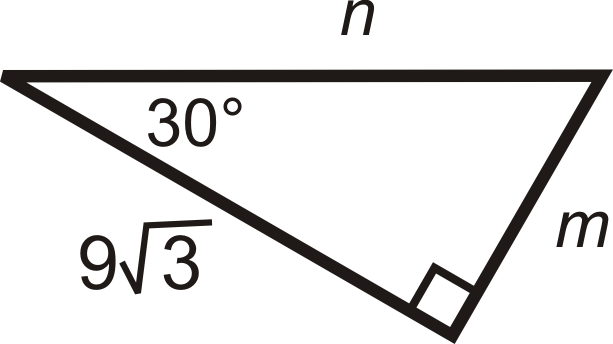# 1.1: 30-60-90 Right Triangles

Hypotenuse equals twice the smallest leg, while the larger leg is $$sqrt{3}$$ times the smallest.

One of the two special right triangles is called a 30-60-90 triangle, after its three angles.

30-60-90 Theorem: If a triangle has angle measures $$30^{\circ}$$, $$60^{\circ}$$ and $$90^{\circ}$$, then the sides are in the ratio $$x: x\sqrt{3}:2x$$.

The shorter leg is always $$x$$, the longer leg is always $$x\sqrt{3}$$, and the hypotenuse is always $$2x$$. If you ever forget these theorems, you can still use the Pythagorean Theorem.

What if you were given a 30-60-90 right triangle and the length of one of its side? How could you figure out the lengths of its other sides?

Example $$\PageIndex{1}$$

Find the value of $$x$$ and $$y$$.Figure $$\PageIndex{1}$$

Solution

We are given the longer leg.

\begin{aligned} x\sqrt{3} &=12 \\ x&=12\sqrt{3}\cdot \dfrac{\sqrt{3}}{\sqrt{3}}=12\dfrac{\sqrt{3}}{3}=4\sqrt{3} \\ &\text{The hypotenuse is} \\ y&=2(4\sqrt{3})=8\sqrt{3} \end{aligned}

Example $$\PageIndex{2}$$

Find the value of $$x$$ and $$y$$.Figure $$\PageIndex{2}$$

Solution

We are given the hypotenuse.

\begin{aligned} 2x&=16 \\ x&=8 \\ \text{The longer leg is} \\ y&=8\cdot \sqrt{3}&=8\sqrt{3} \end{aligned}

Example $$\PageIndex{3}$$

Find the length of the missing sides.Figure $$\PageIndex{3}$$

Solution

We are given the shorter leg. If $$x=5$$, then the longer leg, $$b=5\sqrt{3}$$, and the hypotenuse, $$c=2(5)=10$$.

Example $$\PageIndex{4}$$

Find the length of the missing sides.Figure $$\PageIndex{4}$$

Solution

We are given the hypotenuse. $$2x=20$$, so the shorter leg, $$f=\dfrac{20}{2}=10$$, and the longer leg, $$g=10\sqrt{3}$$.

Example $$\PageIndex{5}$$

A rectangle has sides 4 and $$4\sqrt{3}$$. What is the length of the diagonal?

Solution

If you are not given a picture, draw one.Figure $$\PageIndex{5}$$

The two lengths are $$x$$, $$x\sqrt{3}$$, so the diagonal would be $$2x$$, or $$2(4)=8$$.

If you did not recognize this is a 30-60-90 triangle, you can use the Pythagorean Theorem too.

\begin{aligned} 4^2+(4\sqrt{3})^2&=d^2 \\ 16+48&=d^2 \\ d=\sqrt{64}&=8 \end{aligned}

## Review

1. In a 30-60-90 triangle, if the shorter leg is 5, then the longer leg is __________ and the hypotenuse is ___________.
2. In a 30-60-90 triangle, if the shorter leg is $$x$$, then the longer leg is __________ and the hypotenuse is ___________.
3. A rectangle has sides of length 6 and $$6\sqrt{3}$$. What is the length of the diagonal?
4. Two (opposite) sides of a rectangle are 10 and the diagonal is 20. What is the length of the other two sides?

For questions 5-12, find the lengths of the missing sides. Simplify all radicals.

1.Figure $$\PageIndex{6}$$
2.Figure $$\PageIndex{7}$$
3.Figure $$\PageIndex{8}$$
4.Figure $$\PageIndex{9}$$
5.Figure $$\PageIndex{10}$$
6.Figure $$\PageIndex{11}$$
7.Figure $$\PageIndex{12}$$
8.Figure $$\PageIndex{13}$$

## Vocabulary

Term Definition
30-60-90 Theorem If a triangle has angle measures of 30, 60, and 90 degrees, then the sides are in the ratio $$x : x \sqrt{3} : 2x$$
30-60-90 Triangle A 30-60-90 triangle is a special right triangle with angles of $$30^{\circ}$$, $$60^{\circ}$$, and $$90^{\circ}$$.
Hypotenuse The hypotenuse of a right triangle is the longest side of the right triangle. It is across from the right angle.
Legs of a Right Triangle The legs of a right triangle are the two shorter sides of the right triangle. Legs are adjacent to the right angle.
Pythagorean Theorem The Pythagorean Theorem is a mathematical relationship between the sides of a right triangle, given by $$a^2+b^2=c^2$$, where a and b are legs of the triangle and c is the hypotenuse of the triangle.
Radical The $$\sqrt{}$$, or square root, sign.